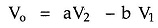# Subtractor or Difference Amplifier

## Subtractor or Difference Amplifier:

Similar to the summer circuit, the subtraction of 2 input voltages is possible with the help of op-amp circuit called subtractor or difference amplifier circuit.

The circuit diagram is shown in the Fig. 2.31.

To find the relation between the inputs and output let us use Superposition principle.Let Vol be the output, with input V1 acting, assuming V2 to be zero. And Vol be the output, with input acting, assuming Vi to be zero.

With V2 zero, the circuit acts as an inverting amplifier. Hence we can write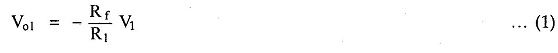While with V1 as zero, the circuit reduces to as shown in the Fig. 2.32.Let potential of node B be VB. The potential of node A is same as B i.e. VA = VB • Applying voltage divider rule to the input V2 loop,.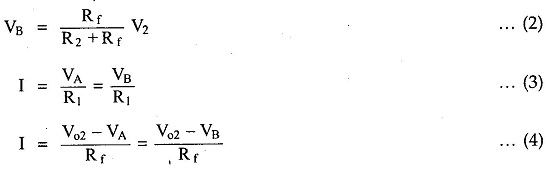Equating the equations (3) and (4),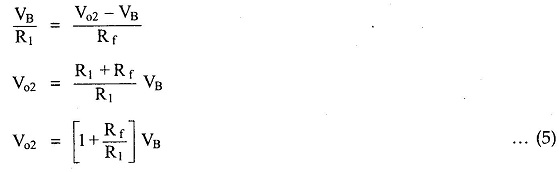Substituting VB from (2) in (5) we get,Hence using Superposition principle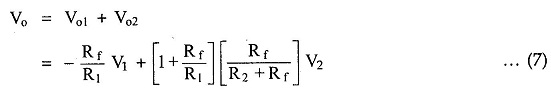Now if the resistances are selected as R1 = R2Thus the output voltage is proportional to the difference between the two input voltages. Thus it acts as a subtractor or difference amplifier.

If Ri = R2 = R f is selected,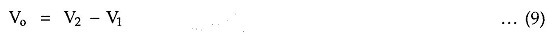But by selecting proper values of R1, R2 and Rf, we can have the subtraction of two inputs with appropriate strengths like